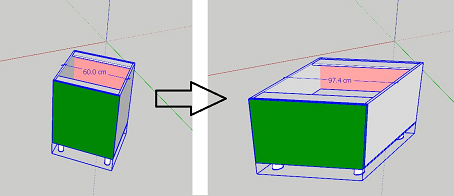# How to scale rotated object?

suppose to we have a box component that orbited 45 degree in Z , so his X and Y axis is not match in world coordinate and this make mistake when i scaled X axis by this code :

``````sele = Sketchup.active_model.selection
origin = sele.transformation.origin
scal = Geom::Transformation.scaling(origin, 2, 1, 1)
sele.transform!(scal)

``````

how can i solve this?

1 Like
``````sele = Sketchup.active_model.selection
origin = sele.transformation.origin

@trr = sele.transformation
sele.transform! @trr.inverse
grpent = sele.entities
grpent.transform_entities(@trr, grpent.to_a)

scal = Geom::Transformation.scaling(origin, 2, 1, 1)
sele.transform!(scal)

grpent = sele.entities
grpent.transform_entities(@trr.inverse, grpent.to_a)
sele.transform! @trr
``````

Hamed, This is a general problem and may happen many times. When you want to write some code for a group or component you’d better move it to the original coordinate and after finish codes return it back.

1 Like

Try this …

``````sele = Sketchup.active_model.selection

# Save the current transformation:
xform = sele.transformation
# Revert the current transformation:
sele.transform!(xform.inverse)

# Create a new compound transformation with scaling:
compound_xform = Geom::Transformation.scaling(xform.origin, 2, 1, 1) * xform

# Apply the compound transformation:
sele.transform!( compound_xform )
``````

@majid866, your code is being applied to the definition’s entities which will effect ALL instances rather that the instance selected.

1 Like

tanks @majid866 i just make a little change in your code as this and solve problem :

``````sele = Sketchup.active_model.selection
origin = sele.transformation.origin

@trr = sele.transformation
sele.transform! @trr.inverse

scal = Geom::Transformation.scaling(ORIGIN, 2, 1, 1)
sele.transform!(scal)
sele.transform! @trr
``````i got it

@DanRathbun this code make thisYea okay then you do them successively as you found out.

Hamed, In the video I run your codes 2 times, first time work well but the second time not. Please be careful about it.

1 Like

Dear Dan, I should learn more from you. I run your code for a box and you can see the result in the video. Would you please let me know about the problem?

1 Like

The problem is that it did not do what I hoped it would. It skewed the instance instead.

So the answer was to apply the scaling transform first, then apply the original transform afterward.
(The original transform was a compound with both rotation and transition.)

1 Like